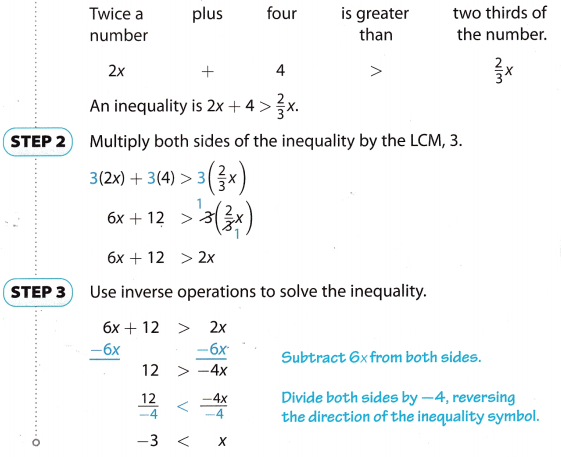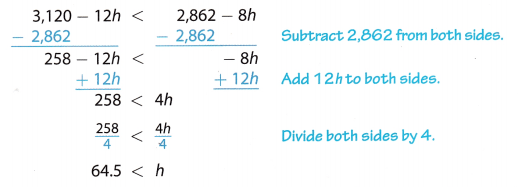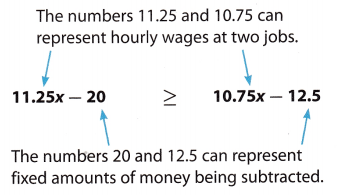# Texas Go Math Grade 8 Lesson 11.4 Answer Key Inequalities with Rational Numbers

Refer to our Texas Go Math Grade 8 Answer Key Pdf to score good marks in the exams. Test yourself by practicing the problems from Texas Go Math Grade 8 Lesson 11.4 Answer Key Inequalities with Rational Numbers.

## Texas Go Math Grade 8 Lesson 11.4 Answer Key Inequalities with Rational Numbers

Example 1

Write an inequality to represent the relationship “Twice a number plus four is greater than two-thirds of the number”. Then solve your inequality.
STEP 1: Write an inequality.Write an inequality to represent the relationship “Three-fourths of a number is greater than five less than the number”. Then solve your inequality.
Consider the number = x
¾ × x ≥ 5 – x
3x/4 ≥ 5-x
3x ≥ 4(5 – x)
3x ≥ 20 – 4x
3x + 4x ≥ 20
7x ≥ 20
x ≥ 2.85
¾ × 2.85 ≥ 5 – 2.85
2.13 < 2.15
2.13 is less than 2.15.
Therefore the three-fourths of a number is not greater than five less than the number.

Example 2

Two water tanks hold 28.62 gallons and 31.2 gallons of water. The larger tank is leaking at a rate of 0.12 gallons per hour. The smaller tank is leaking at a rate of 0.08 gallons per hour. After how many hours will there be less water in the larger tank than in the smaller tank?
STEP 1: Write an inequality. Let h represent the number of hours.
amount in larger tank < amount in smaller tank
31.2 – 0.12h < 28.62 – 0.08h

STEP 2: Multiply both sides of the inequality by 102 = 100.
100(31.2) – 100(0.12h) < 100(28.62) – 100(0.08h)
3,120 – 12h < 2,862 – 8h

STEP 3: Use inverse operations to solve the inequality.So, after 64.5 hours, there will be less water in the larger tank.

Reflect

Question 2.
In Step 2, why do you multiply both sides by 100 rather than by 10?
100(31.2) – 100(0.12h) < 100(28.62) – 100(0.08h)
We can multiply by 100 because on the right side after decimal, there are 2 digits, so you can multiply by 100.
If there is 1 digit in the decimal then multiply by 10.

Question 3.
Bamboo Plant A is 1.2 meters tall and growing at a rate of 0.45 meters per day. Bamboo Plant B is 0.85 meters tall and growing 0.5 meters per day. After how many days will Plant B be taller than Plant A?
Given,
Bamboo Plant A = 1.2 meters tall.
Plant A growing per day = 0.45 meters.
Bamboo Plant B = 0.85 meters tall.
Plant B growing per day = 0.5 meters
Let us consider number of days = d
1.2 + 0.45d < 0.85 + 0.5d
1.2 – 0.85 < 0.5d – 0.45d
0.35 < 0.005d
d < 0.35/0.005
d < 70 days
1.2 + 0.45(70) < 0.85 + 0.5(70)
32.7 < 35.85
Therefore after 70 days will Plant B be taller than Plant A.

Example 3

Write a real-world situation that can be modeled by the inequality
11 .25x – 20 ≥ 1 0.75x – 12.5.
Each side of the inequality consists of a variable term with a constant subtracted from it. The left side must exceed or be equal to the right side.The inequality 11 .25x – 20 ≥ 1 0.75x – 12.5 can represent this situation: Ryan earns $11.25 per hour. His transit cost to and from work is$20 per week. Tony earns $10.75 per hour. His weekly transit cost is$12.50. After how many hours of work in a week do Ryan’s earnings minus transit cost exceed Tony’s earnings minus transit cost?

Write a real-world problem that can be modeled by the inequality
-10 – $$\frac{1}{4}$$x > 20 – $$\frac{1}{2}$$x.
-10 – ¼ x > 20 – 1/2x
-10 – 0.25x > 20 – 0.5x

Write an inequality to represent each relationship. Then solve your inequality. (Example 1)

Question 1.
Three-fourths of a number is less than six plus the number.
Consider the number = x
¾ x = 6 + x
3x/4 = 6 + x
3x = 4(6 + x)
3x = 24 + 4x
3x – 4x = 24
-x = 24
X = – 24
The number = -24
¾ (-24) < 6 +(-24)
-18 = -18
No, three fourth of a number is not less than six plus the number.

Question 2.
One-fifth of a number added to eleven is greater than three-fourths of the number.
Consider the number = x
⅕ x + 11 ≥ ¾ + x
x/5 + 11 ≥ 3x/4
x/5 + 5 × 11/5 ≥ 3x/4.
x+5 ×11 /5 ≥ 3x/4
x+55/5 ≥ 3x/4
20 × x+55/5 ≥ 20 x 3x/4
4(x + 55)≥ 5 x 3x
4x + 220 ≥ 15x
4x≥ 15x – 220
-11x ≥ -220
X ≥ 20.
⅕ x 20 + 11 ≥ ¾ x 20
4 + 11 ≥ 15
15 ≥ 15
No, the one-fifth of a number added to eleven is not greater than three-fourths of the number.

Question 3.
Ian wants to promote his band on the Internet. Site A offers website hosting for $4.95 per month with a$49.95 startup fee. Site B offers website hosting for $9.95 per month with no startup fee. Write and solve an inequality to determine how many months Ian could have his website on Site B and still keep his total cost less than on Site A (Example 2) Answer: Let m represent the number of months Site B ≤ Site A Site B offers website hosting per month with no start-up =$9.95 per month.
Site A offers website hosting per month with start-up fee = $4.95 +$49.95.
$9.95m ≤$4.95m + $49.95 Multiply both sides with 100 100($9.95m) ≤100($4.95m) + 100($49.95)
$995m ≤$495m + $4995$995m – $495m ≤$4995
$500m ≤$4995
m ≤ $4995/$500
m ≤ 9.99 months
9.95(9.99)≤ $4.95(9.99) +$49.95.
99.40 ≤ 99.40
Therefore 9.99 months Lan could have his website on site B.

Question 4.
Write a real-world problem that can be modeled by the inequality 10x > 5.5x + 31.5. (Example 3)
Swetha and Priya invested the same amount in different investment plans. After some period Swetha earned 10 times of that she invested. Priya earned 31.5 more than 5.5 times of his money.
If Swetha earning is more than Priya earning, Then find the amount they invests first time.
Let x be the money.
Swetha earnings = 10x
Priya earnings = 5.5x + 31.5
Swetha earned more than the Priya.
10x > 5.5x + 31.5.

Essential Question Check-In

Question 5.
How can you use inequalities with rational number coefficients and constants to represent real-world problems?
Assume that we have an equation ½ x + 20 ≥ 5/6 x + 12
Ramya gets ½ x per day and she gets a $20 per week and Riya gets 5/6 x per day and she get$12 per week. Find how many days Riya wants to work to reach the Ramya.

Question 6.
Rugs Emporium installs carpet for $80 plus$9.50 per square yard of carpet. Carpets-4-U charges $120 for installation and$7.50 per square yard of carpet. Write and solve an inequality to find the number of square yards of carpet for which Rugs Emporium charges more than Carpets-4-U.
Given,
Rugs Emporium installs carpet for $80 plus$9.50 per square yard of carpet.
Carpets-4-U charges $120 for installation and$7.50 per square yard of carpet.
Consider number of square yards = n
$80 +$9.50n≥ $120 +$7.50n
Multiply both sides with 100
100($80) + 100($9.50n) ≥ 100($120) + 100($7.50n)
$8000 +$950n ≥ $12000 +$750n
$950n –$750n ≥ $12000 –$8000
$200n ≥$4000
n ≥ $4000/$200
n ≥ 20
Therefore number of square yards = 20.

Billie needs to have her refrigerator repaired. She contacts two appliance repair companies and is given the rates shown in the table. Write and solve an inequality to find the number of hours for which Ace’s charge is less than or the same as Acme’s charge.Flat free charges of Acme Repair = 49
Charge per hour of Acme repair = 22.50
Flat free charges of Ace Repair = 0
Charge per hour of Ace repair = 34.75
The inequality equation is
Let us consider the number of hours = h
49 + 22.50h ≤ 0 + 34.75h
49 – 0 ≤ 34.75h – 22.50h
49 ≤ 12.25h
h ≤ 49/12.25
h ≤ 4 hours
49 + 22.50(4) ≤ 0 + 34.75(4).
139 ≤ 139
Therefore, Ace’s charge is the same as Acme’s charge.

Question 8.
Write an inequality with the solution x < 12. The inequality should have the variable on both sides, a decimal coefficient of the variable on the left side, and a decimal anywhere on the right side. One of the decimals should be written in tenths, the other in hundredths.
The solution is
x < 12
2x – x < 1.75 + 10.25
2x – 10.25 < x + 1.75
1.5x -10.25 + 0.5x < 1.5 + x +0.25

Question 9.
Write an inequality with the solution x ≥ 4. The inequality should have the variable on both sides, a fractional coefficient of the variable on the left side, and a fraction anywhere on the right side.
The inequality solution is
x ≥ 4
x ≥ 1.5 + 2.5
2x – x ≥ 3/2 + 5/2
2x – 5/2 ≥ x + 3/2
x – 5/2 ≥ x + 3/2

Question 10.
Multistep According to the Triangle Inequality, the length of the longest side of a triangle must be less than the sum of the lengths of the other two sides. The two shortest sides of a triangle measure $$\frac{3}{10}$$ and x + $$\frac{1}{5}$$, and the longest side measures $$\frac{3}{2}$$x.
a. Write an inequality that uses the Triangle Inequality to relate the three sides.
The length of the longest side of a triangle must be less than the sum of the lengths of the other two sides.
The longest side of the triangle is $$\frac {3}{2}$$ x.
The two shortest sides of a triangle is $$\frac {3}{10}$$ and x + $$\frac {1}{5}$$.
The inequality is
3/2 × x ≤ 3/10 + x + 1/5.

b. For what values of x is the Triangle Inequality true for this triangle?
3/2 × x ≤ 3/10 + x + 1/5.
3x/2 ≤ 0.5 + x
3x ≤ 2(0.5 + x)
3x ≤ 1 + 2x
3x – 2x ≤ 1
x≤ 1
The value of x = 1 is the triangle inequality true for this triangle.

Question 11.
Jose and Maris work for different car dealerships. Jose earns a monthly salary of $3,500 plus a 6% commission on sales. Maris earns a monthly salary of$4,000 plus a 4% commission on sales. Above what value of sales are Jose’s monthly earnings more than those of Maris?
Jose earns a monthly salary = $3,500 + 6% commission on sales. Maris earns a monthly salary =$4,000 + 4% commission on sales.
Maris earns more = $4000 –$3500 = $500. But Joes earns more = 6% – 4% = 2%.$3500 + 0.06n ≥ $4000 + 0.04n 0.06n – 0.04n ≥$4000 – $3500 0.02n ≥$500
n =≥ 500/0.02
n ≥ 5000
$3500 + 0.06(25000) ≥$4000 + 0.04(25000)
$5000 ≥$5000
For the value of n = 25000 values of shares are Jose’s monthly earnings more than or equal to Maris.

Question 12.
Multistep Consider the figures shown.a. Write an expression for the perimeter of each figure.
Perimeter of rectangle = 2(l + w)
length = 0.8n + 0.2
Perimeter of a rectangle = 2(0.8n + 0.2 + n)
= 1.6n + 0.4 + n
Perimeter of a rectangle = 2.6n +0.4
Perimeter of triangle = a + b + c
a = 1.2n + 0.4
b = n + 0.6
c = n
= 1.2n + 0.4 + n +0.6 + n
Perimeter of a triangle = 3.2n + 1

b. For what values of n will the rectangle have a greater perimeter than the triangle?
The perimeter of the rectangle ≥ perimeter of a triangle.
2.6n + 0.4 ≥ 3.2n + 1
2.6n – 3.2n ≥ 1 – 0.4
-0.6n≥ 0.6
n = -1
2.6(-1) + 0.4 ≥ 3.2(-1) + 1
-2.6 + 0.4 ≥ -3.2 + 1
-2.2 ≥ -2.2
If n = 1 the rectangle has a perimeter greater than or equal to the perimeter of the triangle.

H.O.T. Focus on Higher Order Thinking

Question 13.
Draw Conclusions The inequality y < $$\frac{2}{3}$$x + 5 represents all points on the coordinate plane that are below the line y = $$\frac{2}{3}$$x + 5.
a. If you substitute the x- and y-values of the point (6,11) into the inequality, do you get a true statement? What does this mean?
y < $$\frac {2}{3}$$x + 5 equal to
y < ⅔ x + 5.
x = 6
y = 11
11 < ⅔ (11) + 5
11< 12.33
Yes, the given statement is true.

b. If y = 2$$\frac{1}{2}$$, what are the possible values of x? What does this mean?

Question 14.
Communicate Mathematical Ideas Describe what you can do when solving an inequality to ensure that the coefficient of the variable term will be positive.
Answer: If the coefficient of the variable term will be positive. Then multiply or divide the variable with the same coefficient.